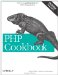# Recipe 2.17. Finding the Distance Between Two Places

#### 2.17.1. Problem

You want to find the distance between two coordinates on planet Earth.

#### 2.17.2. Solution

Use pc_sphere_distance, as shown in Example 2-10.

##### Finding the distance between two points

 ` 2570.18`

#### 2.17.3. Discussion

Since the Earth is not flat, you cannot get an accurate distance between two locations using a standard Pythagorean distance formula. You must use a Great Circle algorithm instead, such as the one in pc_sphere_distance( ).

Pass in the latitude and longitude of your two points as the first four arguments. First come the latitude and longitude of the origin, and then come the latitude and longitude of the destination. The value returned is the distance between them in kilometers:

`// NY, NY (10040) \$lat1 = 40.858704; \$lon1 = -73.928532; // SF, CA (94144) \$lat2 = 37.758434; \$lon2 = -122.435126; \$dist = pc_sphere_distance(\$lat1, \$lon1, \$lat2, \$lon2); printf("%.2f\n", \$dist * 0.621); // Format and convert to miles `

This code finds the distance between New York City and San Francisco, converts the distance to miles, formats it to have two decimal places, and then prints out the result.

Because the Earth is not a perfect sphere, these calculations are somewhat approximate and could have an error up to 0.5%.

pc_sphere_distance( ) accepts an alternative sphere radius as an optional fifth argument. This lets you, for example, discover the distance between points on Mars:

`\$martian_radius = 3397; \$dist = pc_sphere_distance(\$lat1, \$lon1, \$lat2, \$lon2, \$martian_radius); printf("%.2f\n", \$dist * 0.621); // Format and convert to miles `

Recipe 2.12 for trig basics; the Wikipedia entry on Earth Radius at http://en.wikipedia.org/wiki/Earth_radius; and the article "Trip Mapping with PHP" at http://www.onlamp.com/pub/a/php/2002/11/07/php_map.html .PHP Cookbook: Solutions and Examples for PHP Programmers
ISBN: 0596101015
EAN: 2147483647
Year: 2006
Pages: 445

Similar book on Amazon